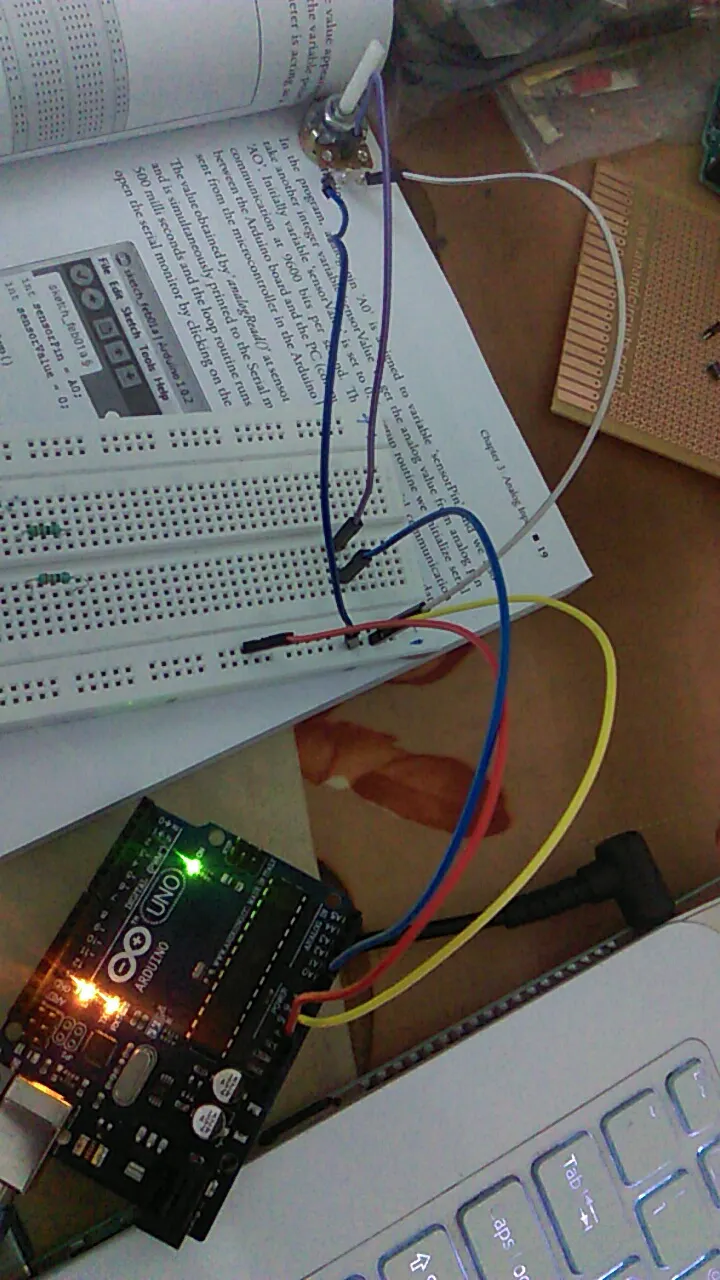Project tutorial# Analog Input Analysis in Arduino Uno Using a Potentiometer © GPL3+

Control the sensor inputs/levels using a potentiometer.

• 53 views
• 2 respects

## Apps and online services

Posted on January 19, 2017 by electromaniaweb

There are six analog input pins in arduino uno namely A0- A5. On the Arduino Mega, we can find sixteen analog pins and eight analog pins on the Arduino Nano. Analog pins are used when we want to read or write variable voltage from 0V to 5V. All the arduino boards consists of 10-bit ADC, i.e. 0V will be read as zero and 5V will be read as 1023 by the Arduino board. All the voltage ranges between 0 and 5 will lie between 0 and 1023.

The potentiometer is used as a voltage divider such that when the knob of the potentiometer is rotated, variable DC voltage can be read by the microcontroller on the Arduino board through any of the analog pins.

### Hardware Required

• Arduino UNO
• Potentiometer

Reading the position of the potentiometer: This project teaches you reading the analog input from the A0 pin and also realizing the in the serial monitor. The central pin of the potentiometer is connected to the analog pin A0 and the both other pins are connected to th +5V power supply and ground respectively. The voltage value to the analog pin A0 is varied as the potentiometer is either rotated clockwise or anti-clockwise. This is how the potentiometer is acting as a “variable voltage sensor."

In the code, analog pin ‘A0’ is assigned to variable sensor pin and we also take another integer variable sensorValue to get the analog value from the analog pin A0. Initially, the sensor value is set to zero. In the setup routine, we initialize serial communication to 9600 bits per second. This opens serial communication between the Arduino board and the laptop, and allows us to view data sent from the microcontroller on the Arduino through the serial monitor.

The value obtained bt analogRead( at sensor pin A0 is placed in variable “sensorValue” and is simultaneously printed to the serial monitor. After this the program passes for 100 milliseconds and the loop routine runs over and over again.

We will get a reading of 0 when the potentiometer is at the minimum position and 1023 when the potentiometer is at the maximum position.

## Code

##### working code.Arduino
```int sensorPin = A0;
int sensorValue = 0;
void setup()
{
Serial.begin(9600);

}

void loop()
{
Serial.printIn(sensorValue);
delay(100);
}
```

#### Published on

December 10, 2018

#### Members who respect this project

See similar projects
you might like

#### An Isolated Analog Input for Arduino

Project tutorial by Giovanni Carrera

• 5,186 views
• 4 respects

#### 2€ OLED I2C Analog Pin Dynamic Plotting

Project showcase by javier muñoz sáez

• 5,668 views
• 12 respects

#### Analog Clock with LED Matrix and Arduino

Project tutorial by LAGSILVA

• 12,391 views
• 40 respects

#### Simple LCD Timer With Arduino UNO

Project showcase by Hugo Blanc

• 10,466 views
• 25 respects

#### Control Arduino Uno Using ESP8266 WiFi Module and Blynk App

Project tutorial by Adithya TG

• 5,258 views